# Standard Form The standard form of any quadratic

• Slides: 23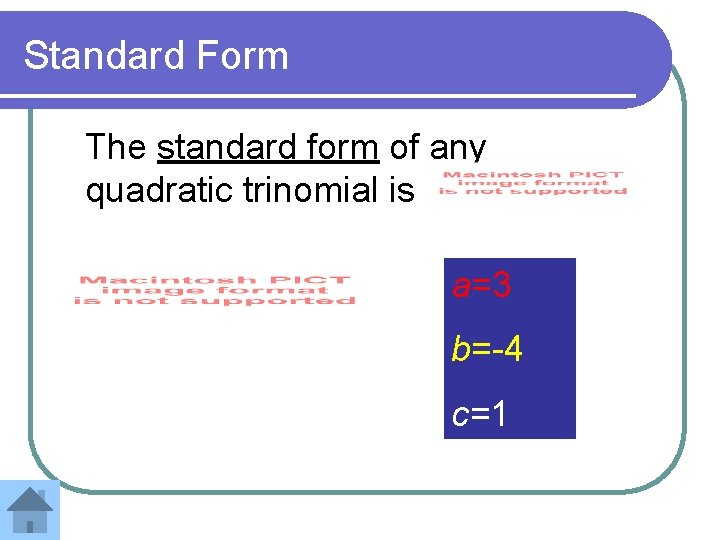Standard Form The standard form of any quadratic trinomial is a=3 b=-4 c=1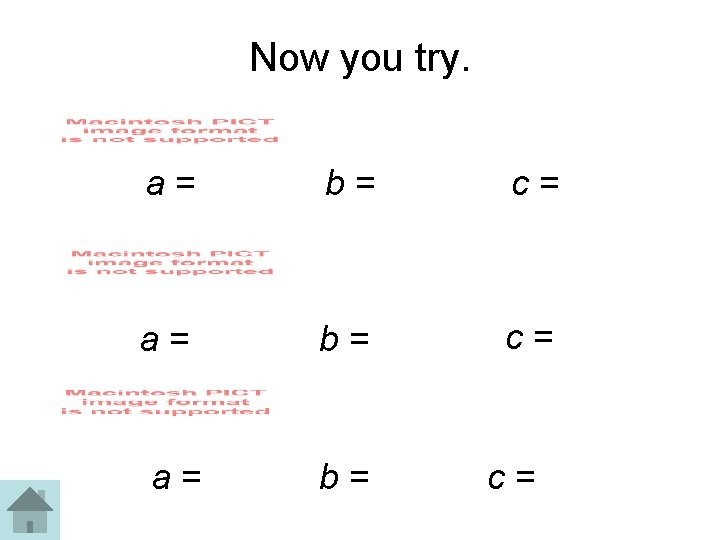Now you try. a= b= c=Factoring when a=1 and c > 0. l First list all the factor pairs of c. l Then find the factors with a sum of l These numbers are used to make the factored expression. 1 , 12 2, 6 3, 4 b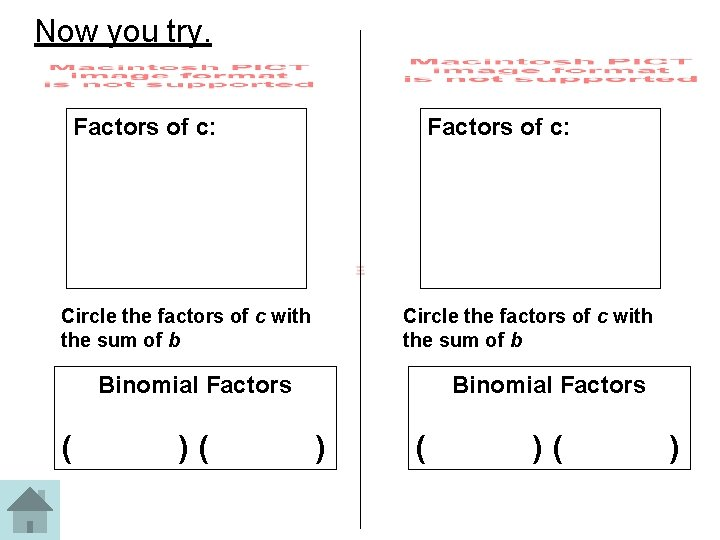Now you try. Factors of c: Circle the factors of c with the sum of b Binomial Factors ( )( Binomial Factors ) ( )( )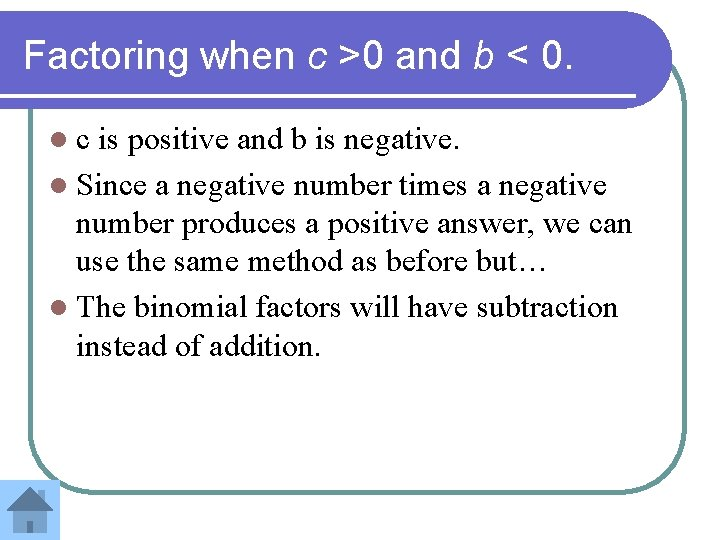Factoring when c >0 and b < 0. lc is positive and b is negative. l Since a negative number times a negative number produces a positive answer, we can use the same method as before but… l The binomial factors will have subtraction instead of addition.Let’s look at First list the factors of 12 1 12 We need a sum of -13 2 6 3 4 Make sure both values are negative!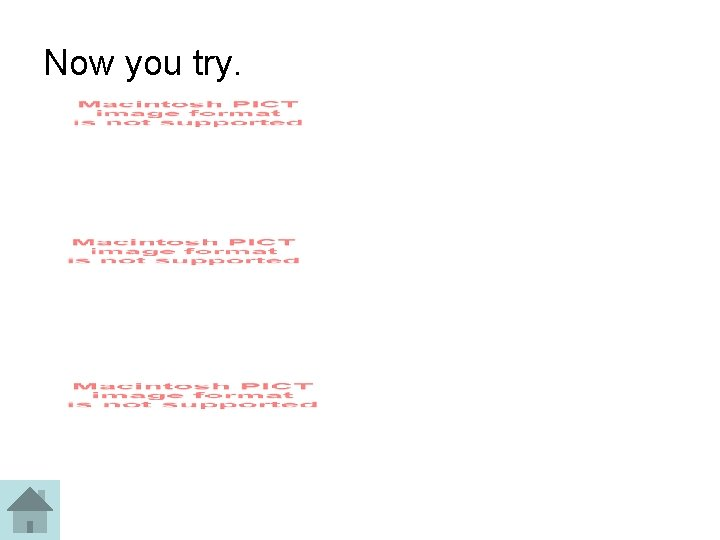Now you try.Factoring when c < 0. We still look for the factors of c. However, in this case, one factor should be positive and the other negative in order to get a negative value for c Remember that the only way we can multiply two numbers and come up with a negative answer, is if one is number is positive and the other is negative!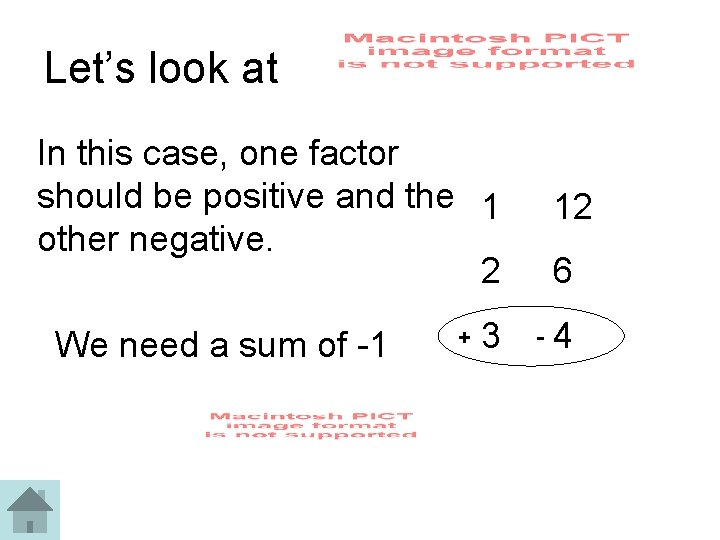Let’s look at In this case, one factor should be positive and the 1 other negative. 2 We need a sum of -1 + 3 12 6 - 4Another Example List the factors of 18. We need a sum of 3 1 18 What factors and signs will we use? 2 9 3 6Now you try. 1. 2. 3. 4.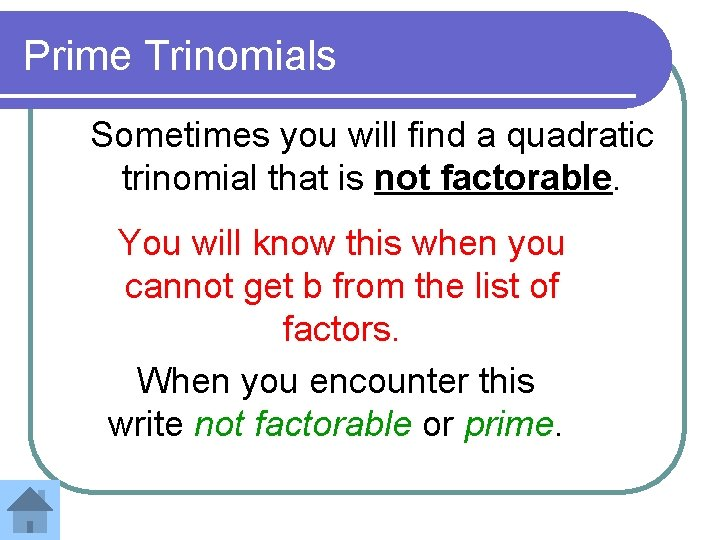Prime Trinomials Sometimes you will find a quadratic trinomial that is not factorable. You will know this when you cannot get b from the list of factors. When you encounter this write not factorable or prime.Here is an example… 1 18 2 9 3 6 Since none of the pairs adds to 3, this trinomial is prime.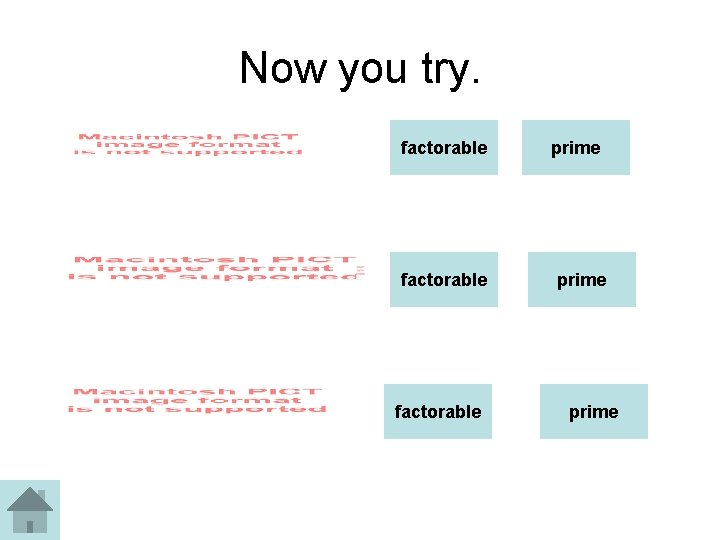Now you try. factorable prime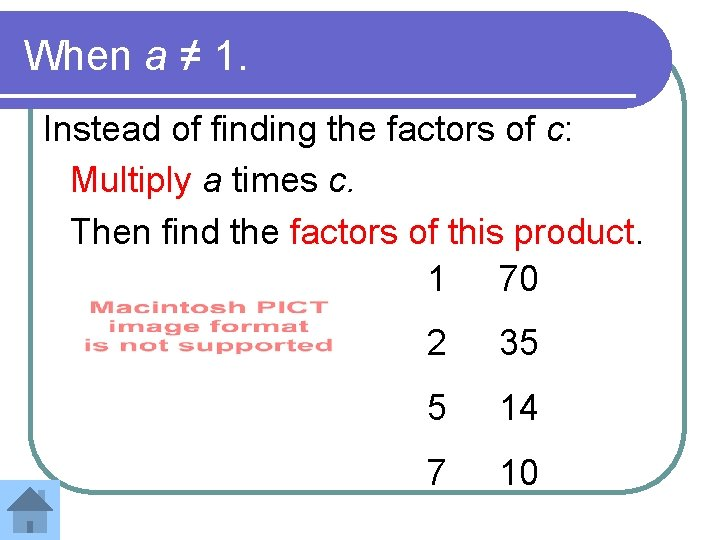When a ≠ 1. Instead of finding the factors of c: Multiply a times c. Then find the factors of this product. 1 70 2 35 5 14 7 10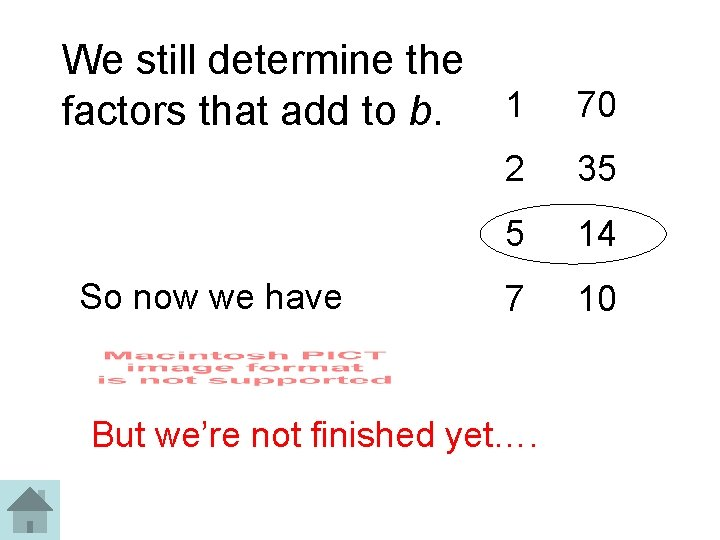We still determine the factors that add to b. 1 70 2 35 5 14 7 10 So now we have But we’re not finished yet….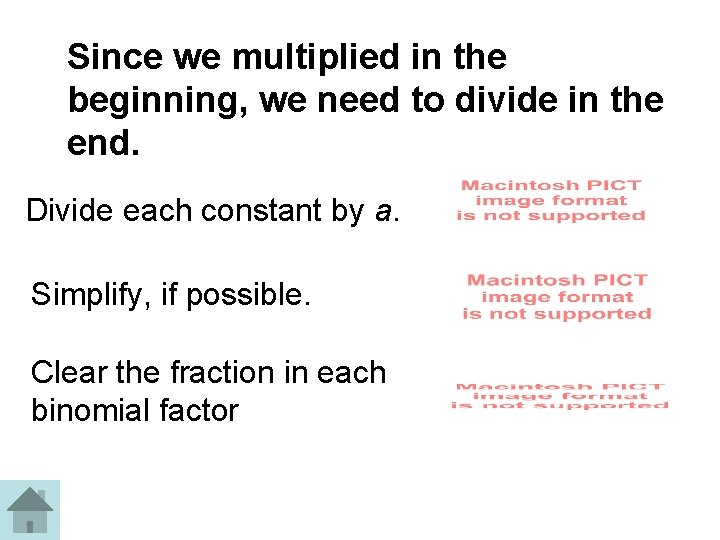Since we multiplied in the beginning, we need to divide in the end. Divide each constant by a. Simplify, if possible. Clear the fraction in each binomial factorRecall • Multiply a times c. • List factors. Look for sum of b • Write 2 binomials using the factors with sum of b • Divide each constant by a. • Simplify, if possible. • Clear the fractions.Now you try.Sometimes there is a GCF. If so, factor it out first. Then use the previous methods to factor the trinomialNow you try. 1. 2.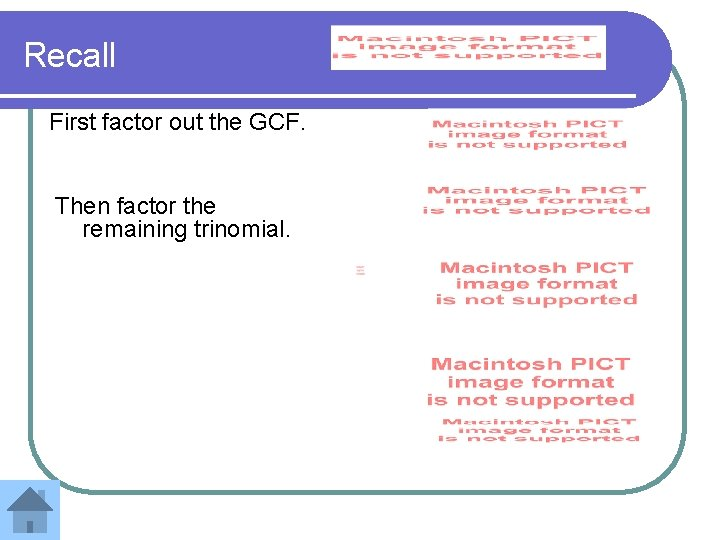Recall First factor out the GCF. Then factor the remaining trinomial.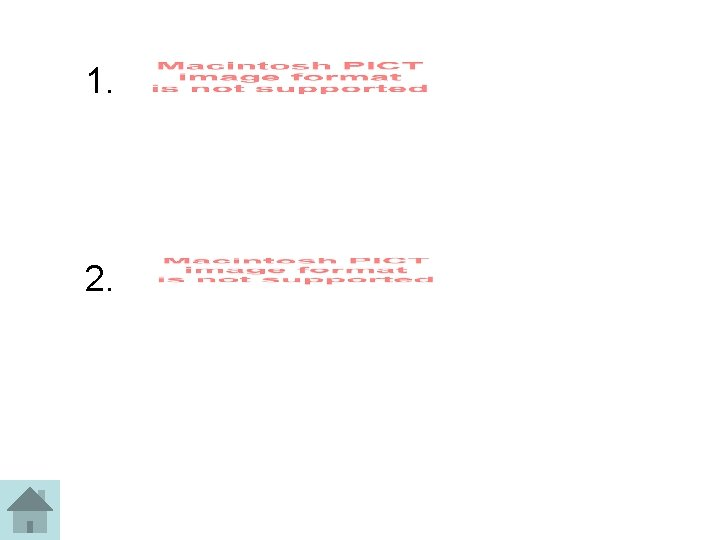1. 2.